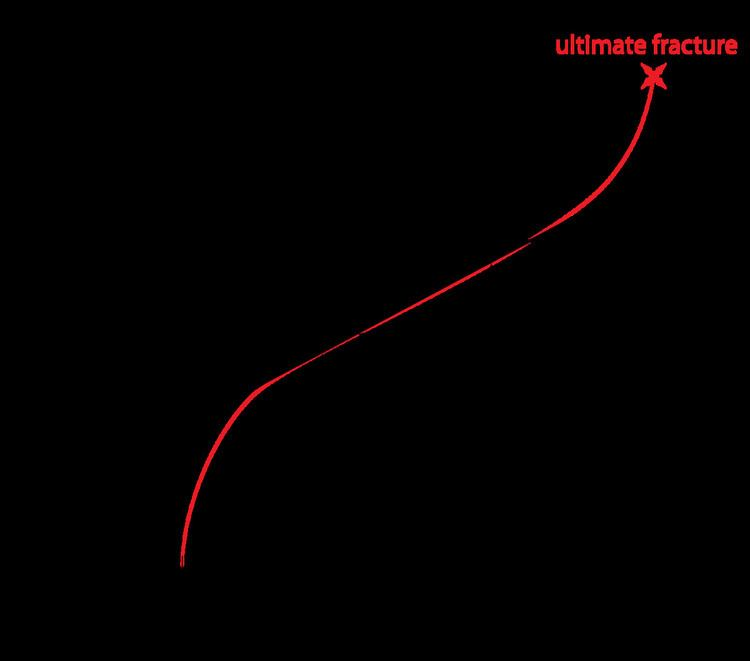# Paris' law

Updated on
Covid-19Paris' law (also known as the Paris-Erdogan law) relates the stress intensity factor range to sub-critical crack growth under a fatigue stress regime. As such, it is the most popular fatigue crack growth model used in materials science and fracture mechanics. The basic formula reads

d a d N = C Δ K m ,

where a is the crack length and N is the number of load cycles. Thus, the term on the left side, known as the crack growth rate, denotes the infinitesimal crack length growth per increasing number of load cycles. On the right hand side, C and m are material constants, and Δ K is the range of the stress intensity factor, i.e., the difference between the stress intensity factor at maximum and minimum loading

Δ K = K m a x K m i n ,

where K m a x is the maximum stress intensity factor and K m i n is the minimum stress intensity factor.

## History and use

The formula was introduced by P.C. Paris in 1961. Being a power law relationship between the crack growth rate during cyclic loading and the range of the stress intensity factor, the Paris law can be visualized as a linear graph on a log-log plot, where the x-axis is denoted by the range of the stress intensity factor and the y-axis is denoted by the crack growth rate.

Paris' law can be used to quantify the residual life (in terms of load cycles) of a specimen given a particular crack size. Defining the stress intensity factor as

K = σ Y π a ,

where σ is a uniform tensile stress perpendicular to the crack plane and Y is a dimensionless parameter that depends on the geometry, the range of the stress intensity factor follows as

Δ K = Δ σ Y π a ,

where Δ σ is the range of cyclic stress amplitude. Y takes the value 1 for a center crack in an infinite sheet. The remaining cycles can be found by substituting this equation in the Paris law

d a d N = C Δ K m = C ( Δ σ Y π a ) m .

For relatively short cracks, Y can be assumed as independent of a and the differential equation can be solved via separation of variables

0 N f d N = a i a c d a C ( Δ σ Y π a ) m = 1 C ( Δ σ Y π ) m a i a c a m 2 d a

and subsequent integration

N f = 2 ( a c 2 m 2 a i 2 m 2 ) ( 2 m ) C ( Δ σ Y π ) m ,

where N f is the remaining number of cycles to fracture, a c is the critical crack length at which instantaneous fracture will occur, and a i is the initial crack length at which fatigue crack growth starts for the given stress range Δ σ . If Y strongly depends on a, numerical methods might be required to find reasonable solutions.

Paris' law Wikipedia

Topics